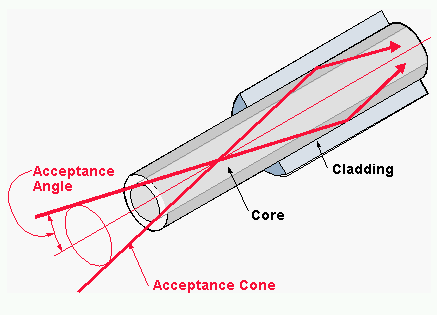numerical aperture

(redirected from Numerical aperture of optical telecommunication fiber)

numerical aperture

[nü′mer·i·kəl ′ap·ə·chər]
(optics)
A measure of the resolving power of a microscope objective, equal to the product of the refractive index of the medium in front of the objective and the sine of the angle between the outermost ray entering the objective and the optical axis. Abbreviated N.A.

numerical aperture

The measurement of the acceptance angle of an optical fiber, which is the maximum angle at which the core of the fiber will take in light that will be contained within the core. Taken from the fiber core axis (center of core), the measurement is the square root of the squared refractive index of the core minus the squared refractive index of the cladding. See critical angle and fiber optics glossary.

The Acceptance AngleThe numerical aperture measures the acceptance angle of a fiber.
Site: Follow: Share:
Open / Close